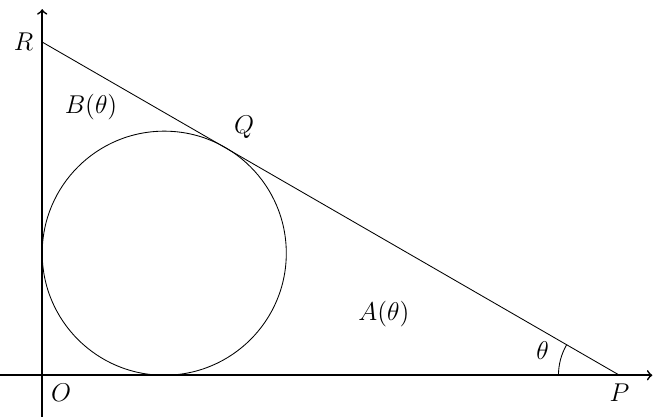CoordinatedGeometry MAT
2007

Problem - 4760

In the diagram below, a line is tangent to a unit circle centered at $Q (1, 1)$ and intersects the two axes at $P$ and $R$, respectively. The angle $\angle{OPR}=\theta$. The area bounded by the circle and the $x-$axis is $A(\theta)$ and the are bounded by the circle and the $y-$axis is $B(\theta)$.

1. Show the coordinates of the point $Q$ is $(1+\sin\theta, 1+\cos\theta)$. Find the equation of line $PQR$ and determine the coordinates of $P$.
2. Explain why $A(\theta)=B\left(\frac{\pi}{2}-\theta\right)$ always holds and calculates $A\left(\frac{\pi}{2}\right)$.
3. Show that $A\left(\frac{\pi}{3}\right)=\sqrt{3}-\frac{\pi}{3}$.The solution for this problem is available for $0.99. You can also purchase a pass for all available solutions for$99.